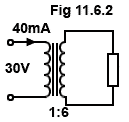# Transformers Quiz

### 1.What is the primary voltage applied to the transformer illustrated in Fig 11.6.1?

### 2.What is the value of current flowing through the resistor R in Fig 11.6.2?

### 3.

Which solution from the following may be used to overcome hysteresis losses in power transformers?

### 4.

Flux linkage between primary and secondary windings of a transformer is proportional to which of the following?

### 5.Refer to the diagram of an autotransformer in Fig. 11.6.3.
If the voltage across A and D is 230V, what will be (approximately) the voltage across A and B?

### 6.

What will be the approximate turns ratio of a transformer matching a microphone of 60Ω impedance to the 47kΩ input impedance of an amplifier?

### 7.

Which of the following would be the most important property of the material, used for an electromagnetic shield around a RF transformer?

### 8.Refer to the diagram of a transformer having a continuously wound, centre tapped secondary winding in Fig. 11.6.4:
What is the relationship between voltages across AB and BC when B is used as the common terminal?

### 9.

Refer to Fig 11.6.4:
What is the voltage between A and C when B is connected to 0V?

### 10.

How are eddy current losses reduced in the cores of RF transformers?